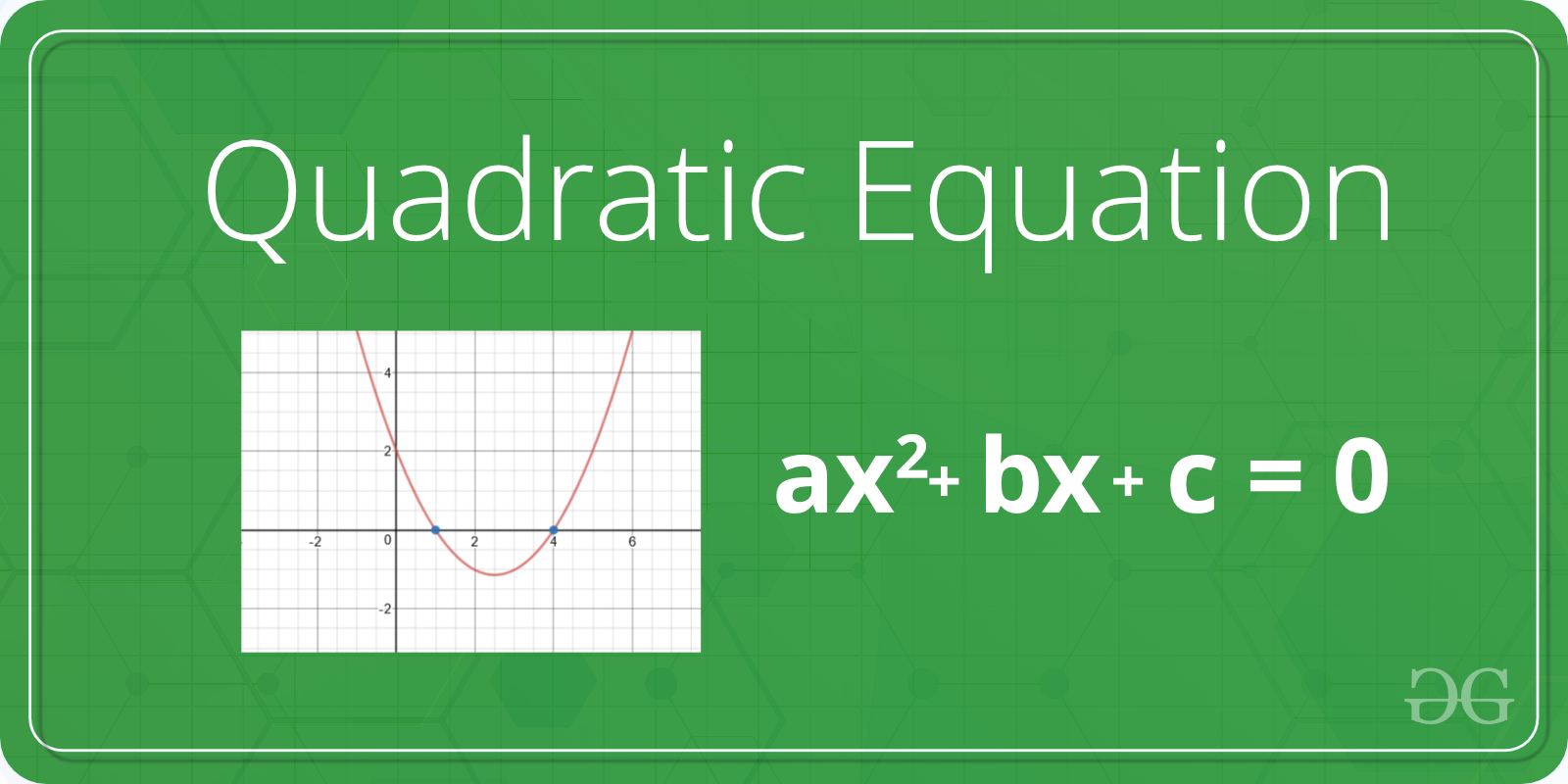Related Articles

# Python program to solve quadratic equation

• Difficulty Level : Basic
• Last Updated : 29 Aug, 2020

Given a quadratic equation the task is solve the equation or find out the roots of the equation. Standard form of quadratic equation is –

ax2 + bx + c
where,
a, b, and c are coefficient and real numbers and also a ≠ 0.
If a is equal to 0 that equation is not valid quadratic equation.Examples:

Input :a = 1, b = 2, c = 1
Output :
Roots are real and same
-1.0

Input :a = 2, b = 2, c = 1
Output :
Roots are complex
-0.5  + i 2.0
-0.5  - i 2.0

Input :a = 1, b = 10, c = -24
Output :
Roots are real and different
2.0
-12.0


Method 1: Using the direct formula

Using the below quadratic formula we can find the root of the quadratic equation.There are following important cases.

If b*b < 4*a*c, then roots are complex
(not real).
For example roots of x2 + x + 1, roots are
-0.5 + i1.73205 and -0.5 - i1.73205

If b*b == 4*a*c, then roots are real
and both roots are same.
For example, roots of x2 - 2x + 1 are 1 and 1

If b*b > 4*a*c, then roots are real
and different.
For example, roots of x2 - 7x - 12 are 3 and 4
 # Python program to find roots of quadratic equationimport math     # function for finding rootsdef equationroots( a, b, c):       # calculating discriminant using formula    dis = b * b - 4 * a * c     sqrt_val = math.sqrt(abs(dis))           # checking condition for discriminant    if dis > 0:         print(" real and different roots ")         print((-b + sqrt_val)/(2 * a))         print((-b - sqrt_val)/(2 * a))           elif dis == 0:         print(" real and same roots")         print(-b / (2 * a))           # when discriminant is less than 0    else:        print("Complex Roots")         print(- b / (2 * a), " + i", sqrt_val)         print(- b / (2 * a), " - i", sqrt_val)   # Driver Program a = 1b = 10c = -24  # If a is 0, then incorrect equationif a == 0:         print("Input correct quadratic equation")   else:    equationroots(a, b, c)

Output:

real and different roots
2.0
-12.0


Method 2: Using the complex math module

First, we have to calculate the discriminant and then find two solution of quadratic equation using cmath module.

 # import complex math moduleimport cmath  a = 1b = 4c = 2  # calculating  the discriminantdis = (b**2) - (4 * a*c)  # find two resultsans1 = (-b-cmath.sqrt(dis))/(2 * a)ans2 = (-b + cmath.sqrt(dis))/(2 * a)  # printing the resultsprint('The roots are')print(ans1)print(ans2)

Output:

The roots are
(-3.414213562373095+0j)
(-0.5857864376269049+0j)


Attention geek! Strengthen your foundations with the Python Programming Foundation Course and learn the basics.

To begin with, your interview preparations Enhance your Data Structures concepts with the Python DS Course. And to begin with your Machine Learning Journey, join the Machine Learning – Basic Level Course

My Personal Notes arrow_drop_up